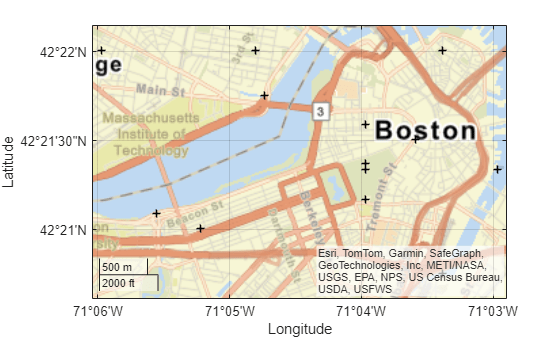# mappointshape

Point shape in planar coordinates

## Description

A `mappointshape` object represents a point or multipoint in planar coordinates. A multipoint is an individual point shape that contains a set of point locations.

To represent a point or multipoint in geographic coordinates, use a `geopointshape` object instead.

## Creation

To create `mappointshape` objects, either:

• Import point data in planar coordinates as a geospatial table using the `readgeotable` function, and then query the `Shape` variable of the table.

• Use the `mappointshape` function (described here).

### Syntax

``shape = mappointshape(x,y)``

### Description

example

````shape = mappointshape(x,y)` creates a `mappointshape` object or array of `mappointshape` objects with the specified x- and y-coordinates. The sizes of `x`, `y`, and the `mappointshape` object array `shape` match.```

### Input Arguments

expand all

x-coordinates, specified as a numeric array or a cell array of numeric arrays.

• Create a point by specifying a scalar, such as `39`.

• Create a multipoint by specifying an array within a cell, such as `{[38 -30 29]}`.

• Create an array of points by specifying an array, such as ```[38 -30 29]```.

• Create an array of points and multipoints by specifying a cell array of numeric arrays, such as `{39,[38 -30 29]}`.

Create placeholders for points with missing data by including `NaN` values. The `NaN` values in `x` must correspond to the `NaN` values in `y`.

The size of `x` must match the size of `y`. For cell arrays, the size of the array in each cell of `x` must match the size of the array in the corresponding cell of `y`.

Data Types: `double` | `cell`

y-coordinates, specified as a numeric array or a cell array of numeric arrays.

• Create a point by specifying a scalar, such as `-113`.

• Create a multipoint by specifying an array within a cell, such as `{[-66 -31 42]}`.

• Create an array of points by specifying an array, such as ```[-66 -31 42]```.

• Create an array of points and multipoints by specifying a cell array of numeric arrays, such as `{-113,[-66 -31 42]}`.

Create placeholders for points with missing data by including `NaN` values. The `NaN` values in `x` must correspond to the `NaN` values in `y`.

The size of `x` must match the size of `y`. For cell arrays, the size of the array in each cell of `x` must match the size of the array in the corresponding cell of `y`.

## Properties

expand all

Number of points, returned as an array of nonnegative integers.

For a `mappointshape` scalar, the value of `NumPoints` is `1` when the `mappointshape` object represents a single point and more than `1` when the object represents a multipoint.

For a `mappointshape` array, the size of `NumPoints` matches the size of the array.

Data Types: `double`

x-coordinates, specified as an array.

For a `mappointshape` scalar, the size of `X` matches the value of `NumPoints`.

For a `mappointshape` array, the size of `X` matches the size of `NumPoints`. If the array contains `mappointshape` objects with multipoints, then accessing the `X` property of the array is not supported. Instead, access the `X` property of individual objects within the array.

This property is read-only for arrays when any element of `NumPoints` is greater than `1`.

`X` and `Y` must be the same size.

Data Types: `double`

y-coordinates, specified as an array.

For a `mappointshape` scalar, the size of `Y` matches the value of `NumPoints`.

For a `mappointshape` array, the size of `Y` matches the size of `NumPoints`. If the array contains `mappointshape` objects with multipoints, then accessing the `Y` property of the array is not supported. Instead, access the `Y` property of individual objects within the array.

This property is read-only for arrays when any element of `NumPoints` is greater than `1`.

`X` and `Y` must be the same size.

Data Types: `double`

Geometric type, returned as `"point"`.

Data Types: `string`

Coordinate system type, returned as `"planar"`.

Data Types: `string`

Projected coordinate reference system (CRS), specified as a `projcrs` object. A projected CRS consists of a geographic CRS and several parameters that are used to transform coordinates to and from the geographic CRS.

## Examples

collapse all

Import a shapefile containing the coordinates of locations in Boston as a geospatial table. The shapefile represents the locations using points. Get information about the points by querying the `Shape` variable of the table.

```GT = readgeotable("boston_placenames.shp"); GT.Shape```
```ans = 13x1 mappointshape array with properties: NumPoints: [13x1 double] X: [13x1 double] Y: [13x1 double] Geometry: "point" CoordinateSystemType: "planar" ProjectedCRS: [1x1 projcrs] ```

Display the locations.

`mapshow(GT)`Create an individual point as a `mappointshape` scalar. Specify the projected CRS as World Equidistant Cylindrical, which has the EPSG authority code `4087`.

```point = mappointshape(-113,39); p = projcrs(4087); point.ProjectedCRS = p```
```point = mappointshape with properties: NumPoints: 1 X: -113 Y: 39 Geometry: "point" CoordinateSystemType: "planar" ProjectedCRS: [1x1 projcrs] ```

Create a multipoint as a `mappointshape` scalar.

```multipoint = mappointshape({[-66 -31 42]},{[38 -30 29]}); multipoint.ProjectedCRS = p```
```multipoint = mappointshape with properties: NumPoints: 3 X: [-66 -31 42] Y: [38 -30 29] Geometry: "point" CoordinateSystemType: "planar" ProjectedCRS: [1x1 projcrs] ```

Create three individual points as a 1-by-3 `mappointshape` array.

```pointVector = mappointshape([-66 -31 42],[38 -30 29]); pointVector.ProjectedCRS = p```
```pointVector = 1x3 mappointshape array with properties: NumPoints: [1 1 1] X: [-66 -31 42] Y: [38 -30 29] Geometry: "point" CoordinateSystemType: "planar" ProjectedCRS: [1x1 projcrs] ```

Create one individual point and one multipoint as a 1-by-2 `mappointshape` array.

```pointMultipoint = mappointshape({-113,[-66 -31 42]},{39, [38 -30 29]}); pointMultipoint.ProjectedCRS = p```
```pointMultipoint = 1x2 mappointshape array with properties: NumPoints: [1 3] Geometry: "point" CoordinateSystemType: "planar" ProjectedCRS: [1x1 projcrs] ```

### Topics

Introduced in R2021b

## SupportGet trial now